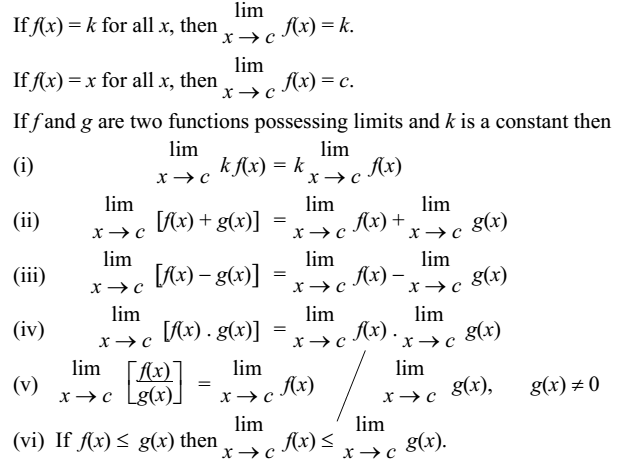# Limit of a Function

The notion of limit is related to the intuitive idea of nearness or closeness. Degree of such closeness cannot be described in terms of basic algebraic operations of addition and multiplication and their inverse operations subtraction and division respectively.

It comes into play in situations where one quantity depends on another varying quantity and you have to know the behavior of the first when the second is very close to a fixed given value.

### Definition

Limit is the value that a function approaches as the input or index approaches some value.

As x → a, f(x) → L, then L is called limit of the function f(x) which is symbolically written as

Limxa f(x) = L

There are two ways x could approach a number a: either from left or from right. All the values of x near a could be less than a or could be greater than a. This leads to two limits – the right hand limit and the left hand limit.

Right hand limit of a function f(x) is that value of f(x) which is dictated by the values of f(x) when x tends to a from the right. Similarly, the left hand limit.

### Existence of Limit

If at any point, left hand limit and right hand limit do not coincide, the the limit of the function at that point does not exist.

### Fundamental Results on Limits